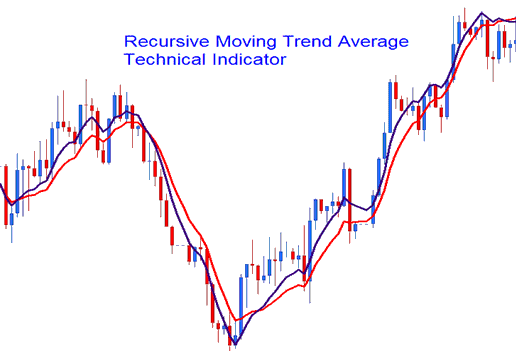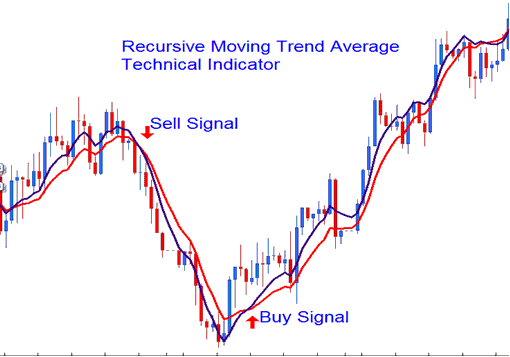# Recursive Moving Trend Average Gold Trading Analysis and Gold Trading Signals

This Indicator is calculated using a mathematical polynomial fit, the formula is referred as the Recursive Moving Polynomial Fit.

This formula used to calculate this indicator only requires a small set of previous data to calculate and predict the next direction of xauusd price movement. The example illustrated and explained below shows two Recursive Averages combined to form a crossover xauusd trading system method.The best technical analysis method is the crossover method where you can combine two recursive averages, such as the 14 and 21. When the two crossovers each other upwards then that is a bullish signal while a downward crossover is a bearish signal.The Recursive Average looks similar to the traditional moving average, the only difference is that is much smoother due to the method of calculation that it uses and much less prone to whipsaws.